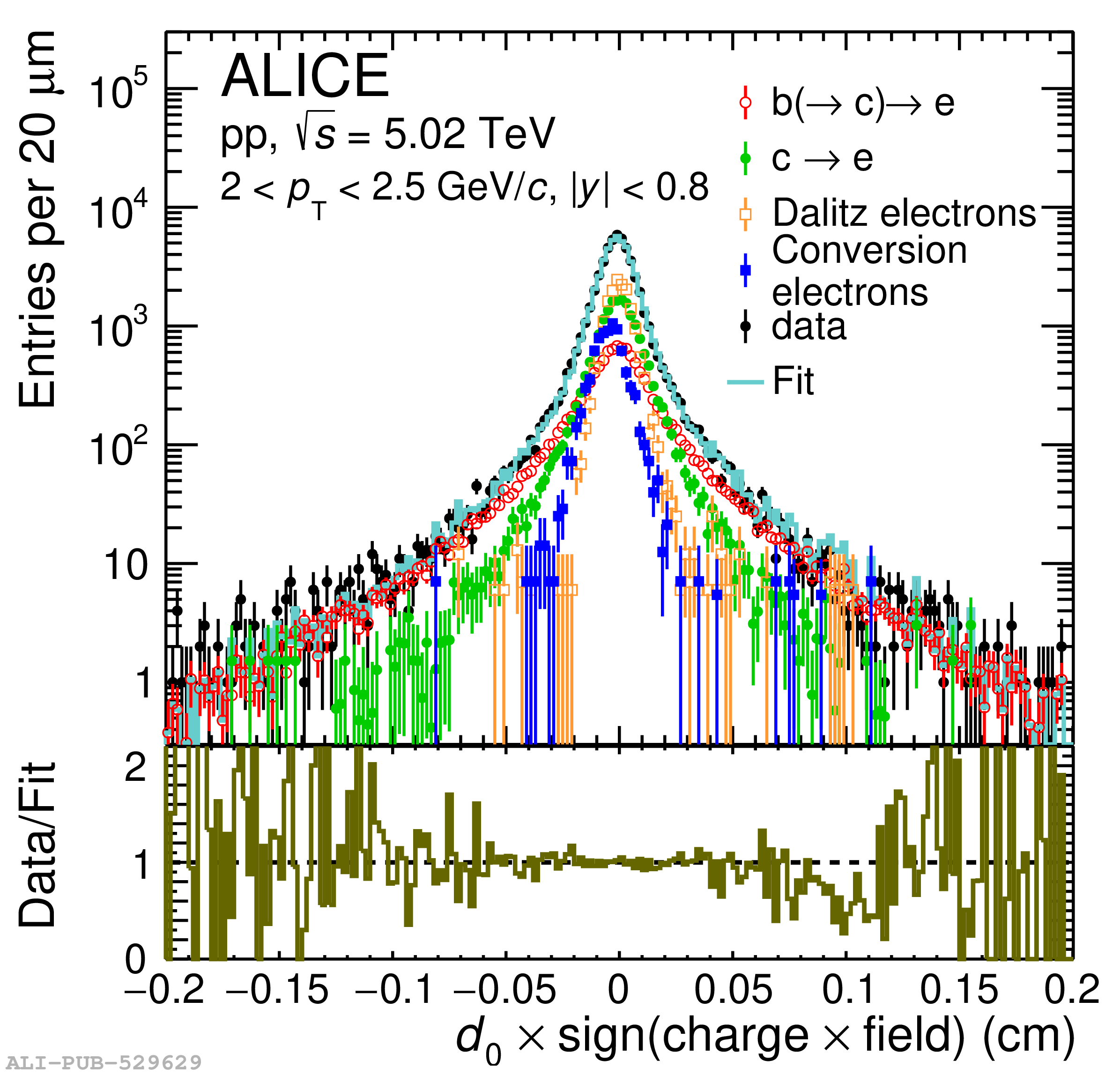# Figure 1

 Examples of the impact parameter fits with the four-template fit approach used in the TPC--TOF analyses. The left panel shows a fit with templates for the four classes of electron sources in pp collisions at $\sqrt{s} = 5.02 {\rm TeV}$ in the lowest $\pt$ interval and the right panel shows a fit example in Pb--Pb collisions at $\sqrt{s_{\rm{NN}}} = 5.02 {\rm TeV}$ in the highest $\pt$ range.